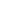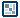## Question of the WeekGravitation
: Physics
written by ilyas usal a.k.a. pom

In this tutorial, we will see how to give an object an effect of gravity.
The original code was provided by Thoriphes.

Note - for an updated version of this tutorial, please visit the Physics-Based Bounce Effect page.

The physics behind it:

We will take the example of a ball free-falling and bouncing against the floor. Basically, what's happening is pretty simple : everything in this world is subject to the gravitation field. In physics, it translates to a force and something we call weight. The force is directed to the ground, and has an influence on the acceleration of objects.

 Force = mass * g g is a constant equal to 10 meters per second per second (m/s2)   acceleration = g   speed = g * t + starting_speed t is the time elapsed since the beginning of the free fall

All this to show you what you already know : wherever the ball will be, it will have a tendency to go down. This implies that when the ball is going down already, it will go down faster, and if it's going up, its speed will decrease to eventually bring it down.

Let's see a first approach of the problem with Flash:

The above code goes inside the Actions panel of the ball, which has to be a movie clip. Think about making a very high window so that you can see the effect.

[ Refresh the page to see the animation ]

What we did is pretty simple : the speed of the ball constantly increases by gravity, so the longer the fall, the faster the ball.

I tried to define a prototype with this function, but I'm having troubles. That's why we'll make a few experiments directly on the parameters. For instance, putting :
speedy = 90 ;
gravity = -2 ;
is an example of reversed gravity with initial speed.

Now we want our ball to bounce against the floor.

In order to do so, we have to set the _y position of the floor. Let's take 500. When the ball bounces, we make the speed change its sign. But we also want it to loose some of its energy. That's why we multiply the speed by bounce, a number < 1 but usually close to 1.
Finally we multiply the speed by -bounce.
We just need to change the code a bit :

[ refresh the page to see the animation ]

You can also define a box, with walls against which the ball will bounce. This is exactly the same technique as what we've just seen : if tests. Note that you have to set speedx to something if you want to see anything.

Now imagine that we want to drag the ball and then let it go.
The first thing we have to do is check if the ball is being dragged. In order to do so, we'll put a button inside the ball movie clip, with the code :

If we're not pressing, we will do with the old code, but if we're pressing, we have to start dragging the movie clip and calculate its speed. Here's the complete code of the movie clip :

If you don't get it, just check the elasticity tutorial, I tried to explain the principle better there.

Last but not least, I told you at the beginning about the physics behind it. Well, it is possible to program the ball so that it moves according to real equations, and not simply ransom figures.
We had speed = g * t + starting_speed. All we have to do is get the time when the ball is thrown. Be careful though : we have to reset the timer and the starting speed every time the ball bounces or is released. Here's the code :

[ the final version of the file ]

The motion isn't really different from the previous one, but it comes directly from the physics equations of motion. There! I hope you understand gravity in Flash a little bit better now.

Click here for all of the source files for this tutorial regarding gravity.

This tutorial is written by Ilyas Usal. Ilyas is also known as ilyaslamasse on the kirupa.com forums! If you have any questions, please post them on the forums by clicking here.

pom